How to Learn in 24 Hours?The Rapid Learning Movie

 Need Help? M-F: 9am-5pm(PST): Toll-Free: (877) RAPID-10 US Direct: (714) 692-2900 Int'l: 001-714-692-2900 24/7 Online Technical Support: The Rapid Support Center Secure Online Order:Need Proof? Testimonials by Our Users

 Rapid Learning Courses: MCAT in 24 Hours (2015-16) USMLE in 24 Hours (Boards) Chemistry in 24 Hours Biology in 24 Hours Physics in 24 Hours Mathematics in 24 Hours Psychology in 24 Hours SAT in 24 Hours ACT in 24 Hours AP in 24 Hours CLEP in 24 Hours DAT in 24 Hours (Dental) OAT in 24 Hours (Optometry) PCAT in 24 Hours (Pharmacy) Nursing Entrance Exams Certification in 24 Hours eBook - Survival Kits Audiobooks (MP3)

 Tell-A-Friend: Have friends taking science and math courses too? Tell them about our rapid learning system.Home »  Mathematics »  Introductory Statistics

Experiment Simulation

Topic Review on "Title":
• Designed experiment, the experimenter imposes one or more experimental conditions on the experimental units and records the response.
• Types of design
• CRD (completely randomized design): an extension of the two independent sample t-test.
• RBD (randomized block design): an extension of the paired difference test.
• AXB factorial experiment: we study two experimental factors and their effect on the response. (we will only discuss CRD and RBD in this tutorial and will not discuss its details in the following slides).
• Formula for CRD ANOVA and Test
• n = bk,
• n = total number of observations
• b = the number of blocks and
• k = the number of treatments within each block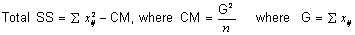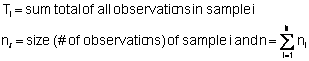Source df SS MS F Treatments k -1 SST SST/(k-1) MST/MSE Error n - k SSE SSE/(n-k) Total n -1 Total SS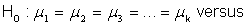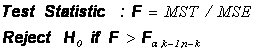•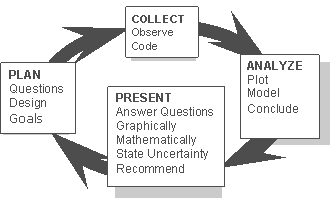Rapid Study Kit for "Title":
 Flash Movie Flash Game Flash Card Core Concept Tutorial Problem Solving Drill Review Cheat Sheet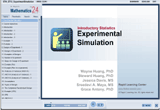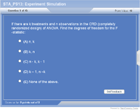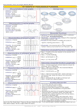"Title" Tutorial Summary : This tutorial shows the basic definitions of by completing this course, you will learn the details about experiment simulation, including introduction, design of experiment, steps in design of experiment, Analysis of Variance (ANOVA), types of experiment, CRD, RBD, axb factorial, etc. Illustrative examples are provided to show how one can use the technique of design of experiment to plan, collect, analyze and present the result of an experiment.

 Tutorial Features: Specific Tutorial Features: Examples showing how different designs and respective ANOVAs are presented. Graphical description of the steps of design experiment is also presented in the tutorial. Series Features: Concept map showing inter-connections of new concepts in this tutorial and those previously introduced. Definition slides introduce terms as they are needed. Visual representation of concepts Animated examples—worked out step by step A concise summary is given at the conclusion of the tutorial.

 "Title" Topic List: Design of experiment Sum of Squares (Total SS, SST, SSE) ANOVA Types of design Step of design of experiment

See all 24 lessons in Introductory Statistics, including concept tutorials, problem drills and cheat sheets:
Teach Yourself Introductory Statistics Visually in 24 Hours